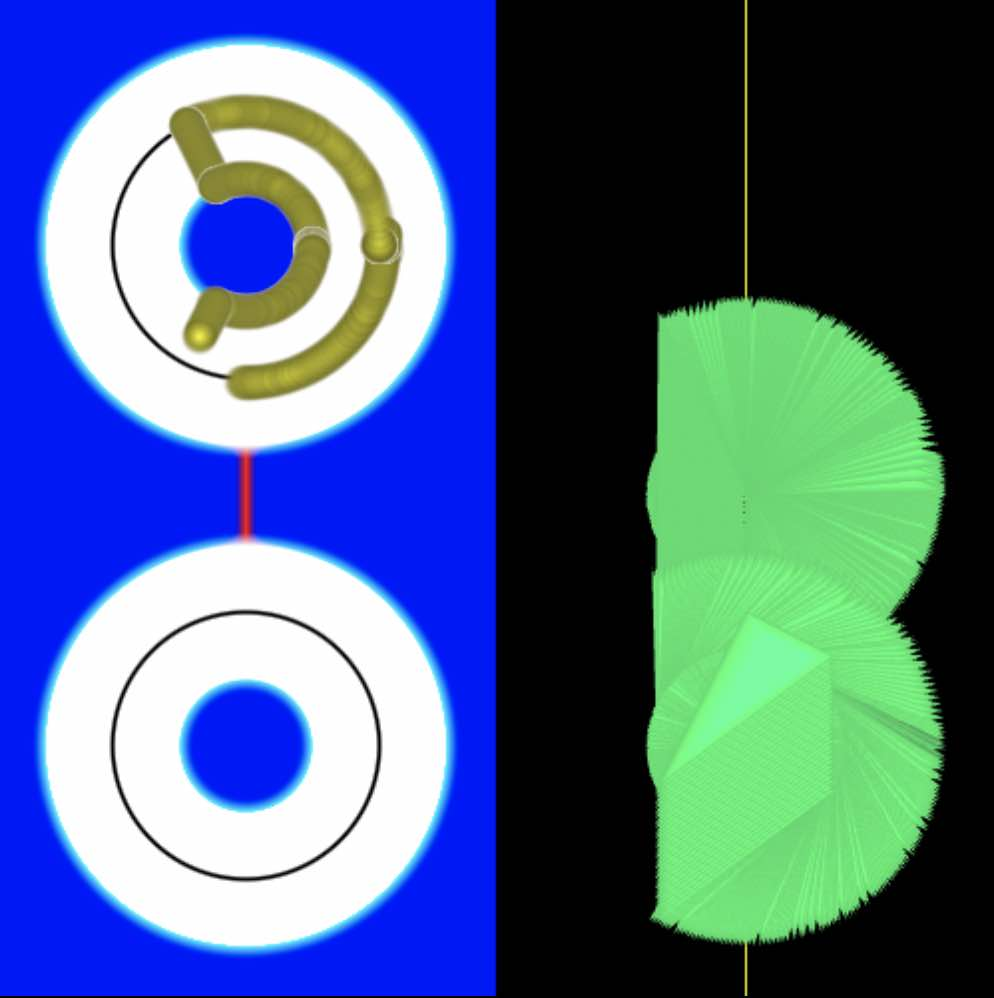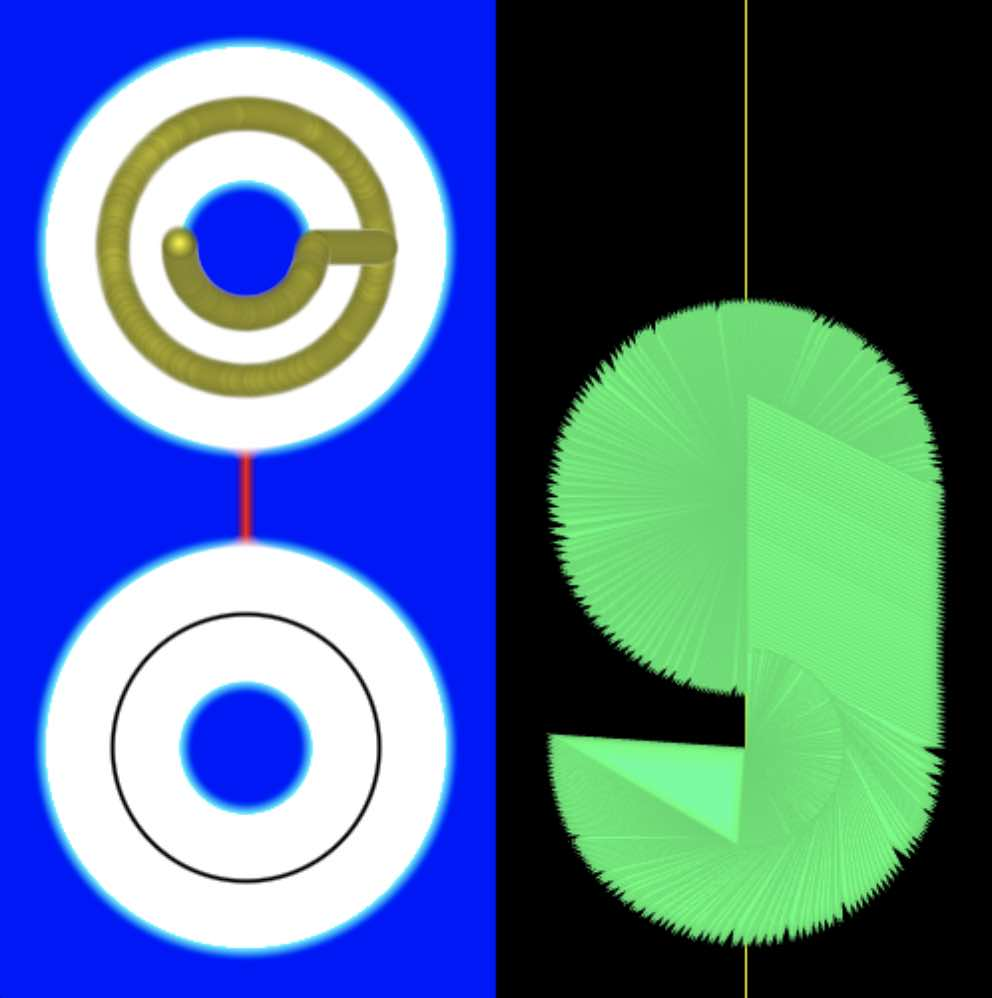## A bit of the space of isometries of the plane

Trail leaving version.
rotation/reflection
Let's parameterise motions (isometries) which are rotations or reflections of R2, but restrict to those that map the origin to some point on the y axis. The left side of the screen shows the point (r,θ,e); e is either 1 (for rotation (upper left)) or -1 (for reflection (lower left)). The dot being on the black circles mean the operation is a linear isometry. The dot being on the red line corresponds to the tranformation not being an isometry. This is not part of the space of isometries of R2, which is disconnected, but I added this little route between the two components. This still lives inside the space of invertible affine maps, except when it squashes the whole thing flat, which we can't avoid.
Note that I'm only showing a piece of a slice of the space of isometries, because it's actually 3 dimensional and infinite, so I can't include it all on the screen easily.
Rotation map is v → M(θ)v + (0,r), where M(θ) means rotation matrix through θ counter clockwise (shown in degrees), and (0,r) is a vector. v is the coordinate vector.
Right side of the screen shows the result of applying the map to the initial triangle.
Not written for touch screens; the yellow dot can be moved via mouse on a computer; otherwise use the sliders. Also need to reload if you change screen size.
Example screen shots: